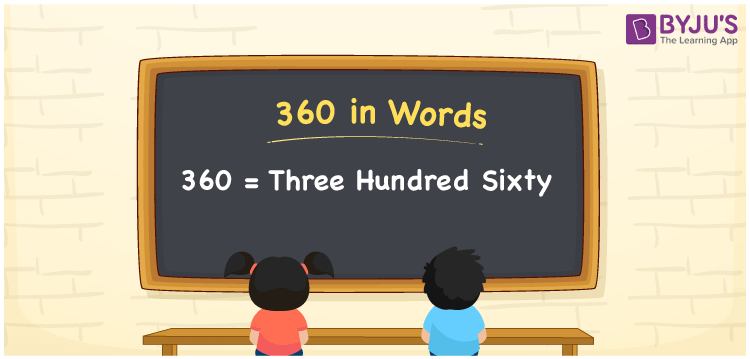# 360 in Words

We can write the number 360 in words as Three hundred sixty. Suppose to read an angle of 360°, and we use the words as “Three hundred sixty degrees”. Similarly, the word form of 360 is used to illustrate the number 360 as a quantity or cost of something. For example, Rs. 360 is read as Three hundred sixty rupees. Also, it is to be noted that 360 is a cardinal number as it represents some amount.

 360 in words Three hundred sixty Three hundred sixty in Numbers 360

## 360 in English Words

We generally use the English alphabet to show numbers in words. Thus, we spell 360 in English words as “Three hundred sixty”.## How to Write 360 in Words?

The number 360 has three digits. Hence, we need to make a place value chart with three columns as shown below.

 Hundreds Tens Ones 3 6 0

Here, ones = 0, tens = 6, hundreds = 3

The above values can be expanded as:

3 × Hundred + 6 × Ten + 0 × One

= 3 × 100 + 6 × 10

= 300 + 60

= Three hundred + Sixty

= Three hundred sixty

Therefore, 360 in words = Three hundred sixty

We know that 360 is a natural number that precedes 361 and succeeds 359.

360 in words – Three hundred sixty

Is 360 an even number? – Yes

Is 360 an odd number? – No

Is 360 a composite number? – Yes

Is 360 a prime number? – No

Is 360 a perfect square number? – No

Is 360 a perfect cube number? – No

## Frequently Asked Questions on 360 in Words

### How do you write 360 in English words?

In English words, we write the number 360 as Three hundred sixty.

### What is the value of 220 + 140 in words?

220 + 140 = 360 Thus, the value of 220 + 140, i.e. 360 in words three hundred sixty.

### How to write Rs. 360 in words on a cheque?

On a cheque, the amount Rs. 360 can be written in words as “Three hundred sixty rupees only”.